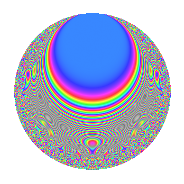# Properties

 Label 210.3.qLevel 210 Weight 3 Character orbit q Rep. character $$\chi_{210}(149,\cdot)$$ Character field $$\Q(\zeta_{6})$$ Dimension 64 Newform subspaces 1 Sturm bound 144 Trace bound 0

# Related objects

## Defining parameters

 Level: $$N$$ $$=$$ $$210 = 2 \cdot 3 \cdot 5 \cdot 7$$ Weight: $$k$$ $$=$$ $$3$$ Character orbit: $$[\chi]$$ $$=$$ 210.q (of order $$6$$ and degree $$2$$) Character conductor: $$\operatorname{cond}(\chi)$$ $$=$$ $$105$$ Character field: $$\Q(\zeta_{6})$$ Newform subspaces: $$1$$ Sturm bound: $$144$$ Trace bound: $$0$$

## Dimensions

The following table gives the dimensions of various subspaces of $$M_{3}(210, [\chi])$$.

Total New Old
Modular forms 208 64 144
Cusp forms 176 64 112
Eisenstein series 32 0 32

## Trace form

 $$64q - 64q^{4} + 8q^{6} - 4q^{9} + O(q^{10})$$ $$64q - 64q^{4} + 8q^{6} - 4q^{9} - 8q^{10} + 4q^{15} - 128q^{16} + 8q^{19} - 88q^{21} - 8q^{24} + 12q^{25} - 8q^{30} + 152q^{31} + 16q^{36} - 208q^{39} - 16q^{40} + 106q^{45} - 56q^{46} - 64q^{49} - 140q^{51} - 56q^{54} + 616q^{55} - 4q^{60} + 104q^{61} + 512q^{64} - 160q^{66} + 456q^{69} - 144q^{70} + 298q^{75} - 32q^{76} - 360q^{79} + 304q^{81} - 80q^{84} - 408q^{85} - 688q^{90} - 288q^{91} + 240q^{94} - 16q^{96} - 568q^{99} + O(q^{100})$$

## Decomposition of $$S_{3}^{\mathrm{new}}(210, [\chi])$$ into newform subspaces

Label Dim. $$A$$ Field CM Traces $q$-expansion
$$a_2$$ $$a_3$$ $$a_5$$ $$a_7$$
210.3.q.a $$64$$ $$5.722$$ None $$0$$ $$0$$ $$0$$ $$0$$

## Decomposition of $$S_{3}^{\mathrm{old}}(210, [\chi])$$ into lower level spaces

$$S_{3}^{\mathrm{old}}(210, [\chi]) \cong$$ $$S_{3}^{\mathrm{new}}(105, [\chi])$$$$^{\oplus 2}$$

## Hecke characteristic polynomials

There are no characteristic polynomials of Hecke operators in the database